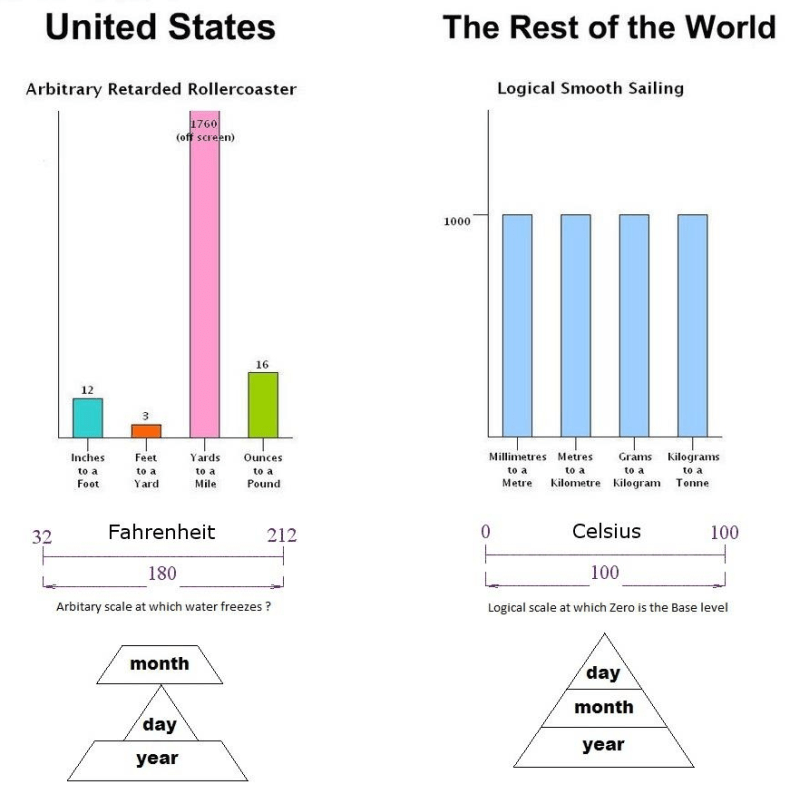Life..

## 1 pound feathers vs. 1 pound gold

In school everybody (at least where I went to school) learned how important it is to mark your answers and numbers with the corresponding unit of measurement, especially in physics, but also a little in math. This mainly to avoid confusion, and also it worked as a fine check to see if you had calculated correctly. If the answer should be given in Newton, and your answer was in kg, it probably went wrong somewhere in your calculations.

As I grew up in Norway, where the metric system (or more specifically, the SI units) is used, I usually just don’t get why people insist on using other systems, like the system of measurements they use in the US. Many of you have probably seen this image:One of the theories of how Fahrenheit made his temperature scale is that he set 0°F (−17,8°C) as the lowest temperature he could get by mixing a right amound of salt and crushed ice, and 100°F (37,8°C) as approximately the body temperature of a human being.. What?

Now to the main point of this blog post. This question has circulated the internet for long while now, and at first glance it seems way too simple of a question, but if you actually think about it, it could be more complex than you think. Consider the following question:

Which is heavier; A pound of feathers, or a pound of gold?

If you would have asked the relation between a kg feathers and a kg gold, the answer would have been simple – they weigh the same, because 1 kg is 1 kg, which is one of the benefits of the SI units.

But what is the problem then? Well, a pound is an ambiguous unit of mass. The main thing to notice is that the question asks about feathers and gold. In the US, Avoirdupois weight is the most commonly used, and if the measuring system of the US wasn’t confusing enough, they actually use a different weight for measuring precious metals (Troy weight). The Avoirdupois weight is the one shown in the image above, where 16 ounces equals 1 pound. However, one Troy ounce equals 192/175 Avoirdupois ounces, and one Troy pound is only 12 Troy ounces..

«Converted» to gram (g): 1 Avoirdupois pound = 453.6 g, 1 Troy pound = 373.2 g.

So a pound of feathers is actually heavier than a pound of gold!

Reklamer